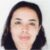Disclosure: This post may contain affiliate links, meaning when you click the links and make a purchase, we receive a commission.

# How to Use the FORMULATEXT Function in Excel

The FORMULATEXT Function is a new Excel function, which was introduced in Excel 2013 and later versions of Excel. Using the FORMULATEXT Function will allow you to select any cell containing a formula and have it returned as a text string, in another cell. This can be useful if you want to analyze the formulas in your worksheet alongside their results.

The syntax for the FORMULATEXT Function is:

=FORMULATEXT(reference)

where reference is a cell or a range of cells.

So, let’s get started with a simple example to illustrate how to show formulas in a worksheet and then how to use this specific function.

## Introduction

We have a worksheet containing Sales Data of three hypothetical sales people and formulas calculating the maximum, minimum, average and utilized for other calculations in the worksheet.## Showing All the Formulas in the Worksheet Using Show Formulas

1) We could display all the formulas in the worksheet by going to Formulas>Formula Auditing>Show Formulas.2) This returns the results shown below, and all the formulas of the worksheet in each of the cells containing them are shown.3) Going to Formulas>Formula Auditing>Show Formulas or by pressing CTRL+` on the keyboard, toggles the formulas off and returns the worksheet back to the standard view.

Read More: How to Use Compound Interest Formula in Excel

## Using the F2 key to show a specific formula in a cell

1) One can also click in a specific cell containing a formula, for example, in this worksheet, select cell F18 as shown below.2) While in the cell press the F2 key on the keyboard in order to see the formula.3) Pressing ESC restores the cell back to the Ready mode and out of Editing Mode.

## Using the FORMULATEXT Function

1) In order to have the formula in a cell delivered as a text string in another cell, you would have to use the FORMULATEXT Function.

2) We have the highest sales amount of the three salespeople shown in cell E20, so in cell E21 we are going to use the FORMULATEXT Function to show the formula and thus we type:

=FORMULATEXT(E20)3) Upon pressing CTRL-ENTER =MAX(B18, F18, J18) is returned to the cell as a text string, as shown below.And there you have it.

HowToUseTheFORMULATEXTFunctionInExcel

## Conclusion

The FORMULATEXT Function delivers formulas in Excel cells, in text strings. This can be a good way of annotating your worksheet with the formulas used while learning for an Excel exam or Finance exam, as well as a method of analyzing the formulas in the workbook alongside their actual results.

Please feel free to comment and tell us if you use the FORMULATEXT Function frequently.

Taryn is a Microsoft Certified Professional, who has used Office Applications such as Excel and Access extensively, in her interdisciplinary academic career and work experience. She has a background in biochemistry, Geographical Information Systems (GIS) and biofuels. She enjoys showcasing the functionality of Excel in various disciplines.

1. ReplyHenk June 22, 2017 at 8:23 PM

Hi Taryn,
I am curious, why do you always use ctrl-enter instead of just enter?
Regards

• ReplyJune 28, 2017 at 5:18 AM

Hi Henk thanks for the comment, the reason I use CTRL-ENTER is because after pressing CTRL-ENTER one stays in the cell that contains the actual formula, whereas pressing ENTER takes you to one cell below the actual formula, TAB of course takes one to one cell right of the cell containing the formula. So its a preference on my part basically :-). It would actually be interesting to see what the preferences of general Excel users are – and if its related to anything else – maybe ambidextrous people for example, prefer for the most part using the TAB key. So yes one can use ENTER or TAB instead of CTRL-ENTER but I like staying in the cell that contains the actual formula.:-)

2. ReplyMike November 27, 2017 at 10:30 PM

I too had been wondering about your use of Ctrl-Enter – Thanks for the explanation!! I’ll have to give it a go.

3. ReplyDecember 16, 2017 at 10:16 PM

Hi Mike thanks for the comment :-). Yes definitely and see which one of the three you prefer using.

I am wondering now if there is some other variable that is related to whether one prefers using TAB, ENTER or CTRL-ENTER in Excel. I am right-handed so I am wondering if it (my preference) maybe is related to that, or something else entirely.

It would also be interesting to see the results of a poll of Excel users and which of the three turned out to be the most popular.